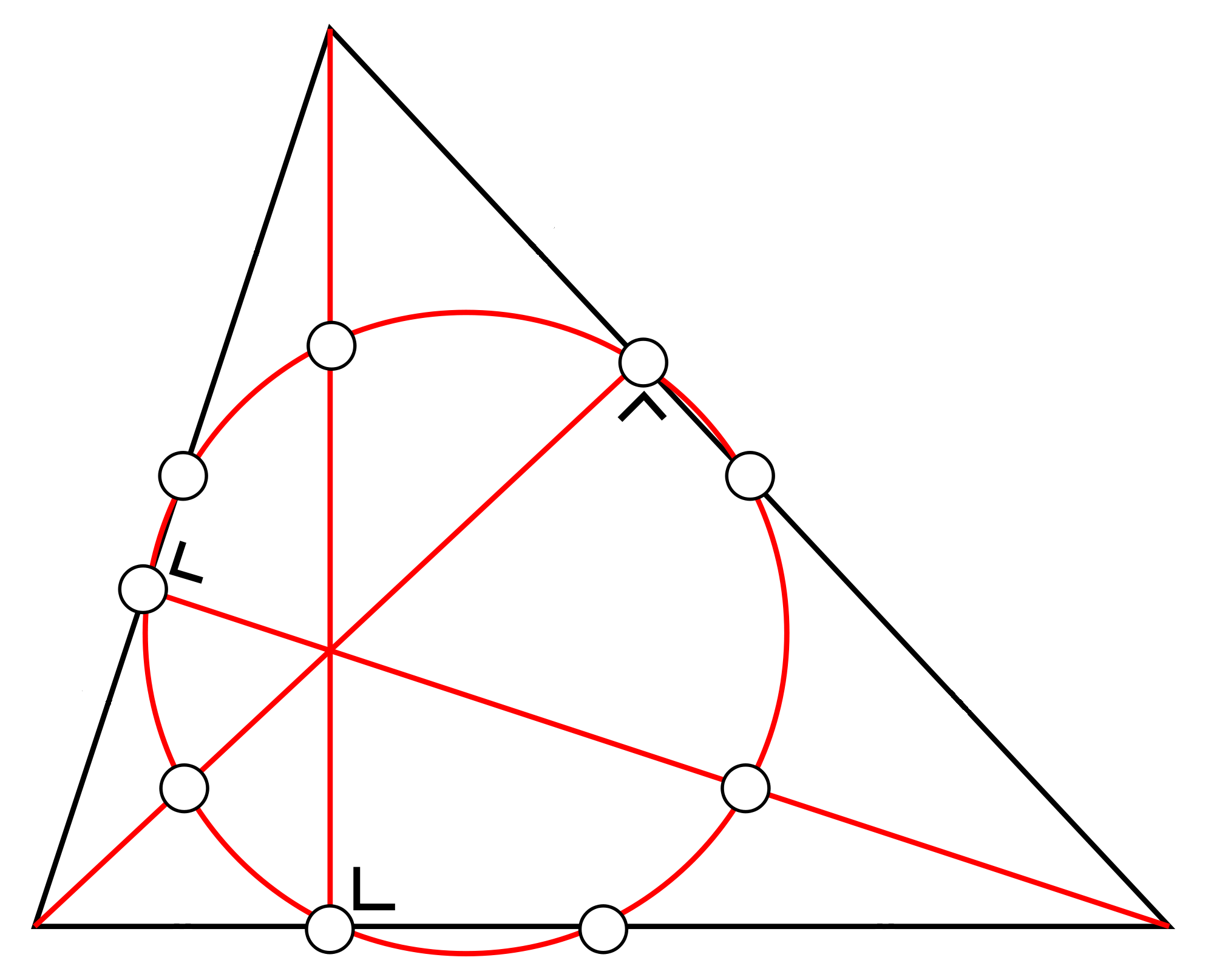# The nine-point-circle

For a triangle with sides 13, 15 and altitude 12, find the radius of the circle that passes through the following points:

• the midpoint of each side,
• the foot of each altitude, and
• the midpoint of the line segment from each vertex to the orthocenter.

If the radius can be written as $a + \frac{m}{n}$, where $a,m,$ and $n$ are positive integers, $m and $m$ and $n$ are coprime, find $a+m+n$.

Note: Assume the given altitude to be through the vertex common to both the given sides.×